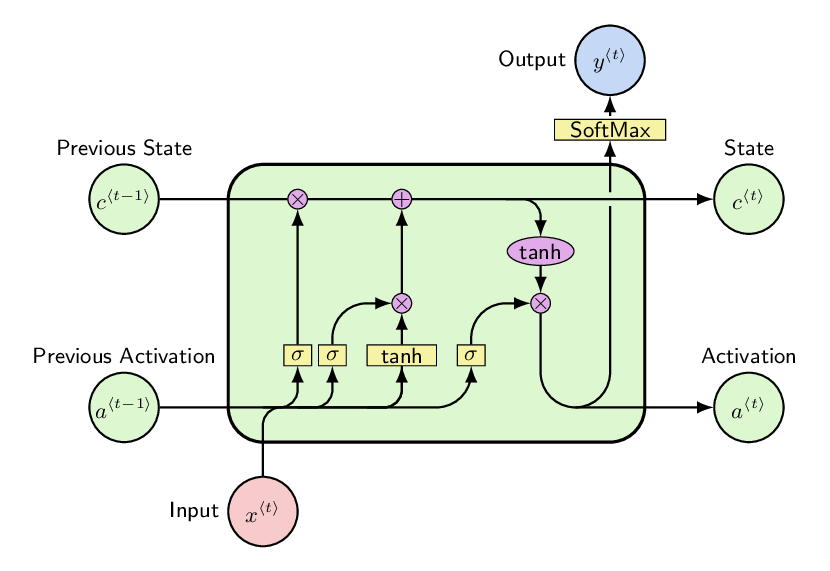# Rumours Detection using Neural Network based Model

Original article can be found here (source): Deep Learning on MediumImporting Libraries

`import numpy as npimport pandas as pdfrom collections import defaultdictimport seaborn as snsimport kerasfrom keras.preprocessing.text import Tokenizerfrom keras.preprocessing.sequence import pad_sequencesfrom keras.utils.np_utils import to_categoricalfrom keras.layers import Embeddingfrom keras.layers import Dense, Input, Flattenfrom keras.layers import Conv1D, MaxPooling1D, Dropoutfrom keras.models import Modelfrom keras.models import Sequentialfrom keras.layers.normalization import BatchNormalizationfrom matplotlib import pyplot as pltfrom keras.layers import LSTM, GRUfrom sklearn.model_selection import train_test_split`

Data Preprocessing

In preprocessing part, we remove all hashtags, user names and web links using Python library preprocessor. We then use tokenizer for splitting sentences to word tokens, padding sentences to ensure that all sentences in a list have the same length and converting labels to one-hot vectors.

`import preprocessor as pdef clean_str(string): string = re.sub(r"\\", "", string)  string = re.sub(r"\'", "", string)  string = re.sub(r"\"", "", string)  return string.strip().lower()data_train = pd.read_csv('../twitter_training_dataset_a.csv')list_labels = list(set(data_train.labels))texts = []labels = []for i in range(data_train.text.shape): text = p.clean(str(data_train.text[i])) texts.append(text) labels.append(data_train.labels[i])tokenizer = Tokenizer(num_words=MAX_NB_WORDS)tokenizer.fit_on_texts(texts)sequences = tokenizer.texts_to_sequences(texts)word_index = tokenizer.word_indexdata = pad_sequences(sequences, maxlen=MAX_SEQUENCE_LENGTH)labels = to_categorical(np.asarray(labels),num_classes = len(list_labels))`

Splitting data into training and testing set

Shuffling data and splitting it into training and testing set with test size of 20%.

`indices = np.arange(data.shape)np.random.shuffle(indices)data = data[indices]labels = labels[indices]x_train, x_test, y_train, y_test = train_test_split( data, labels, test_size=0.20, random_state=42)`

Using pre-trained word embeddings

For word embeddings, we used a pre-trained word-embedded vector – Glove. Glove is a word-embedded vector which contains around 400000 words mapped with 100-dimensional vector each.

`embeddings_index = {}with open('../../glove.6B.100d.txt') as f: for line in f: values = line.split() word = values coefs = np.asarray(values[1:], dtype='float32') embeddings_index[word] = coefsembedding_matrix = np.random.random((len(word_index) + 1, 100))for word, i in word_index.items(): embedding_vector = embeddings_index.get(word) if embedding_vector is not None: embedding_matrix[i] = embedding_vectorembedding_layer = Embedding(len(word_index) + 1, 100, weights=[embedding_matrix], input_length=MAX_SEQUENCE_LENGTH)`

Building the model

The model contains pre-trained embedding layer followed by a Conv1D layer with kernel size of 5, a LSTM layer with 100 nodes with dropout of 20%, 3 dense layers with 128, 64, 32 nodes respectively and the output layer (4 nodes for Task A, or 3 nodes for Task B). The model was trained on training set for 50 epochs with batch size of 128.

`model = Sequential()model.add(embedding_layer)model.add(Conv1D(filters=32, kernel_size=5, padding='same', activation='relu'))model.add(MaxPooling1D(pool_size=2))model.add(LSTM(100, dropout=0.2, recurrent_dropout=0.2))model.add(BatchNormalization())model.add(Dense(128, activation='relu'))model.add(Dense(64, activation='relu'))model.add(Dense(32, activation='relu'))model.add(Dense(len(list_labels), activation='softmax'))model.compile(loss='binary_crossentropy', optimizer='adam', metrics=['accuracy'])model.fit(x_train, y_train, epochs=50, batch_size=128)`

Testing the model

The model got an accuracy of 47.57% and 42.86% with loss of 1.204 and 0.453 for Subtask A and Subtask B respectively.